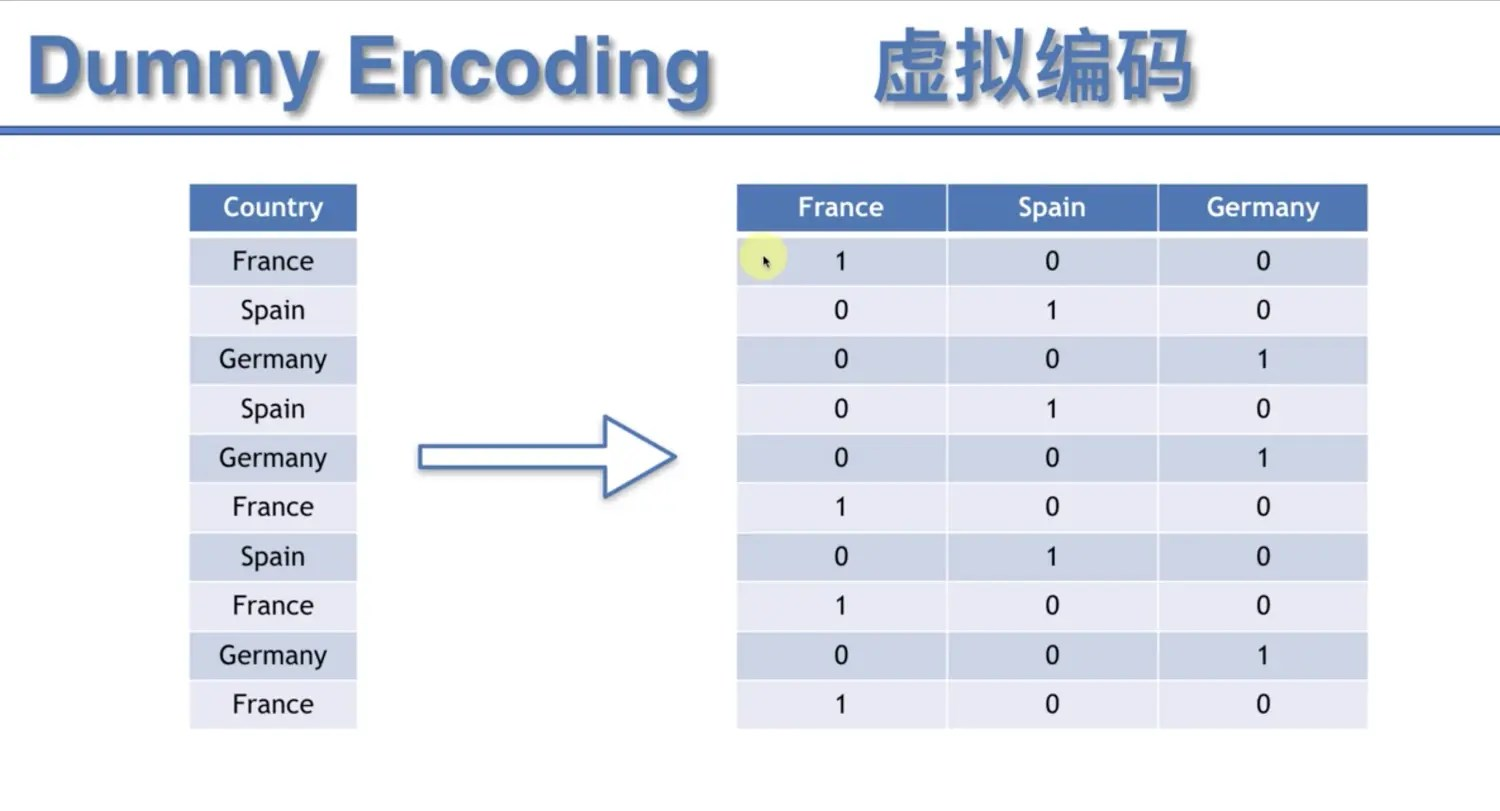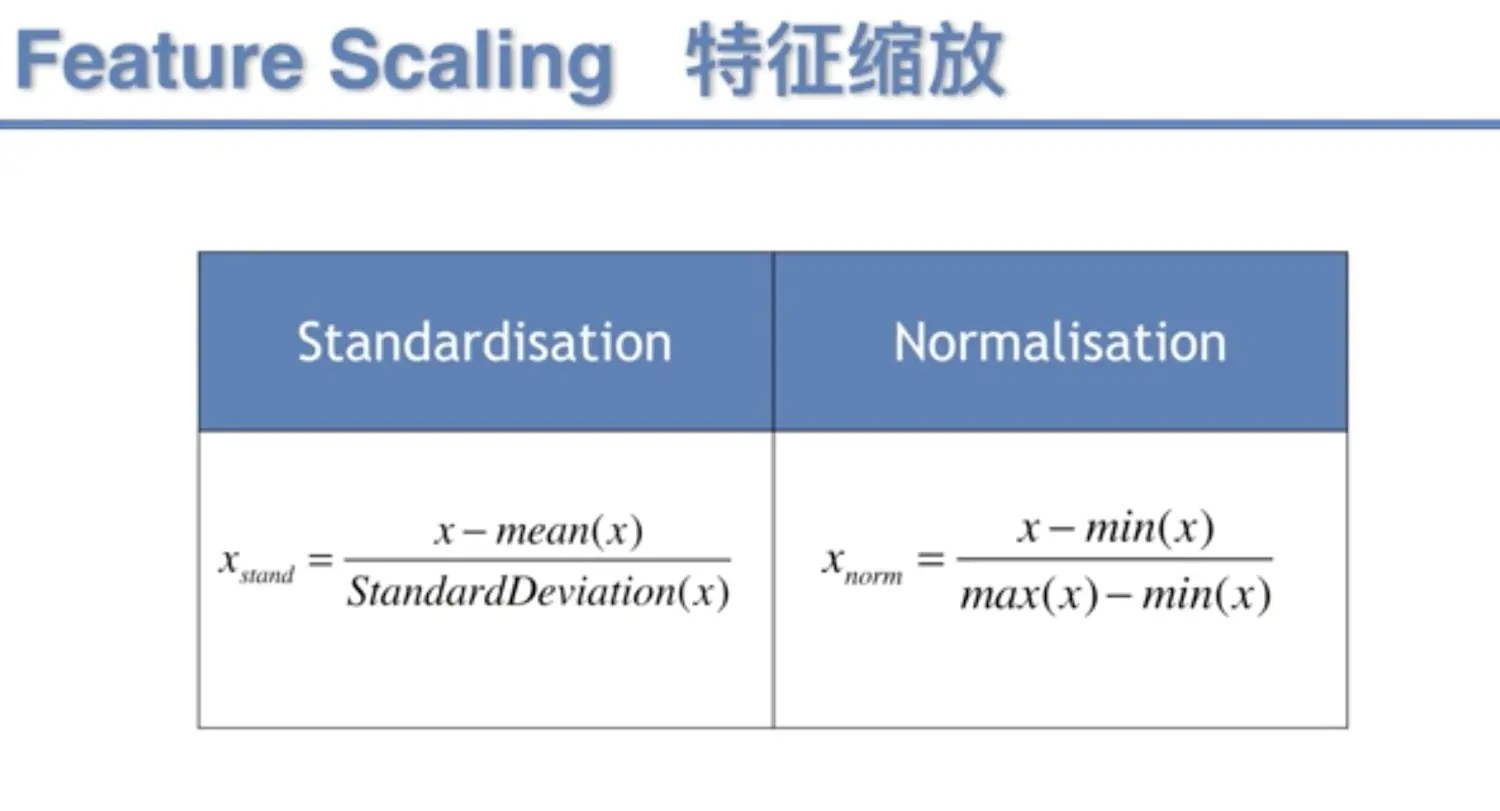# 机器学习A-Z～数据预处理

1. 导入数据集
2. 处理缺失数据
3. 分类数据
4. 数据分成训练集和测试集
5. 特征缩放

## 导入数据集

Country,Age,Salary,Purchased
France,44,72000,No
Spain,27,48000,Yes
Germany,30,54000,No
Spain,38,61000,No
Germany,40,,Yes
France,35,58000,Yes
Spain,,52000,No
France,48,79000,Yes
Germany,50,83000,No
France,37,67000,Yes


import pandas as pd
from sklearn.model_selection import train_test_split
from sklearn.preprocessing import StandardScaler
data_path = '../data/Data.csv'

#import the dataset
X = dataset.iloc[:,:-1].values
y = dataset.iloc[:,3].values


X为数据中所有行的除了最后一列的所有数据，y则是最后一列的结果。这样，数据集就被导入进来了。

## 处理缺失数据

• 删除缺失的数据（操作简单但风险很大，容易删除重要的数据）
• 取该列的平均值来代替缺失数据

# Taking care of missing data
from sklearn.preprocessing import Imputer
imputer = Imputer(missing_values = 'NaN', strategy = 'mean', axis = 0)
imputer = imputer.fit(X[:, 1:3])
X[:, 1:3] = imputer.transform(X[:, 1:3])


## 分类数据

# Encoding categorical data
# Encoding the Independent Variable
from sklearn.preprocessing import LabelEncoder, OneHotEncoder
labelencoder_X = LabelEncoder()
X[:, 0] = labelencoder_X.fit_transform(X[:, 0])
onehotencoder = OneHotEncoder(categorical_features = )
X = onehotencoder.fit_transform(X).toarray()
# Encoding the Dependent Variable
labelencoder_y = LabelEncoder()
y = labelencoder_y.fit_transform(y)## 数据分成训练集和测试集

#spliting the dataset to trainset and testset
X_train, X_test, y_train, y_test = train_test_split(X, y, test_size = 0.2, random_state = 0)


## 特征缩放#feature scaling
sc_X = StandardScaler()
X_train = sc_X.fit_transform(X_train)
X_test = sc_X.transform(X_test)